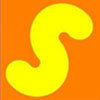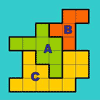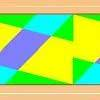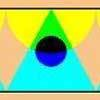# Resources tagged with: Dynamic geometry

Filter by: Content type:
Age range:
Challenge level:

There are 24 NRICH Mathematical resources connected to Dynamic geometry, you may find related items under Physical and Digital Manipulatives.

Broad Topics > Physical and Digital Manipulatives > Dynamic geometry### The Line and Its Strange Pair

##### Age 14 to 16 Challenge Level:

In the diagram the point P' can move to different places along the dotted line. Each position P' takes will fix a corresponding position for P. If P' moves along a straight line what does P do ?### Trapezium Four

##### Age 14 to 16 Challenge Level:

The diagonals of a trapezium divide it into four parts. Can you create a trapezium where three of those parts are equal in area?##### Age 14 to 16 Challenge Level:##### Age 14 to 16 Challenge Level:

Four rods are hinged at their ends to form a convex quadrilateral. Investigate the different shapes that the quadrilateral can take. Be patient this problem may be slow to load.### Using Geogebra

##### Age 11 to 18### Eight Ratios

##### Age 14 to 16 Challenge Level:

Two perpendicular lines lie across each other and the end points are joined to form a quadrilateral. Eight ratios are defined, three are given but five need to be found.### Mapping the Wandering Circle

##### Age 14 to 16 Challenge Level:

In the diagram the point P can move to different places around the dotted circle. Each position P takes will fix a corresponding position for P'. As P moves around on that circle what will P' do?### Points in Pairs

##### Age 14 to 16 Challenge Level:

Move the point P to see how P' moves. Then use your insights to calculate a missing length.### Two Shapes & Printer Ink

##### Age 14 to 16 Challenge Level:

If I print this page which shape will require the more yellow ink?### The Rescaled Map

##### Age 14 to 16 Challenge Level:

We use statistics to give ourselves an informed view on a subject of interest. This problem explores how to scale countries on a map to represent characteristics other than land area.### Rotations Are Not Single Round Here

##### Age 14 to 16 Challenge Level:

I noticed this about streamers that have rotation symmetry : if there was one centre of rotation there always seems to be a second centre that also worked. Can you find a design that has only. . . .### One Reflection Implies Another

##### Age 14 to 16 Challenge Level:

When a strip has vertical symmetry there always seems to be a second place where a mirror line could go. Perhaps you can find a design that has only one mirror line across it. Or, if you thought that. . . .### A Roll of Patterned Paper

##### Age 14 to 16 Challenge Level:

A design is repeated endlessly along a line - rather like a stream of paper coming off a roll. Make a strip that matches itself after rotation, or after reflection### Secants Interactivity

##### Age 14 to 16 Challenge Level:

Move the ends of the lines at points B and D around the circle and find the relationship between the length of the line segments PA, PB, PC, and PD. The length of each of the line segments is. . . .### Napoleon's Theorem

##### Age 14 to 18 Challenge Level:

Triangle ABC has equilateral triangles drawn on its edges. Points P, Q and R are the centres of the equilateral triangles. What can you prove about the triangle PQR?### Three Balls

##### Age 14 to 16 Challenge Level:

A circle has centre O and angle POR = angle QOR. Construct tangents at P and Q meeting at T. Draw a circle with diameter OT. Do P and Q lie inside, or on, or outside this circle?### The Medieval Octagon

##### Age 14 to 16 Challenge Level:

Medieval stonemasons used a method to construct octagons using ruler and compasses... Is the octagon regular? Proof please.### Isosceles

##### Age 11 to 14 Challenge Level:

Prove that a triangle with sides of length 5, 5 and 6 has the same area as a triangle with sides of length 5, 5 and 8. Find other pairs of non-congruent isosceles triangles which have equal areas.##### Age 14 to 16 Challenge Level:

The points P, Q, R and S are the midpoints of the edges of a non-convex quadrilateral.What do you notice about the quadrilateral PQRS and its area?### Triangle Incircle Iteration

##### Age 14 to 16 Challenge Level:

Keep constructing triangles in the incircle of the previous triangle. What happens?### Napoleon's Hat

##### Age 16 to 18 Challenge Level:

Three equilateral triangles ABC, AYX and XZB are drawn with the point X a moveable point on AB. The points P, Q and R are the centres of the three triangles. What can you say about triangle PQR?### Cushion Ball

##### Age 16 to 18 Challenge Level:

The shortest path between any two points on a snooker table is the straight line between them but what if the ball must bounce off one wall, or 2 walls, or 3 walls?### Polycircles

##### Age 14 to 16 Challenge Level:

Show that for any triangle it is always possible to construct 3 touching circles with centres at the vertices. Is it possible to construct touching circles centred at the vertices of any polygon?### Fixing It

##### Age 16 to 18 Challenge Level:

A and B are two fixed points on a circle and RS is a variable diamater. What is the locus of the intersection P of AR and BS?